# Quadratic Word Problems Maxmin Finding Roots Recap You

• Slides: 37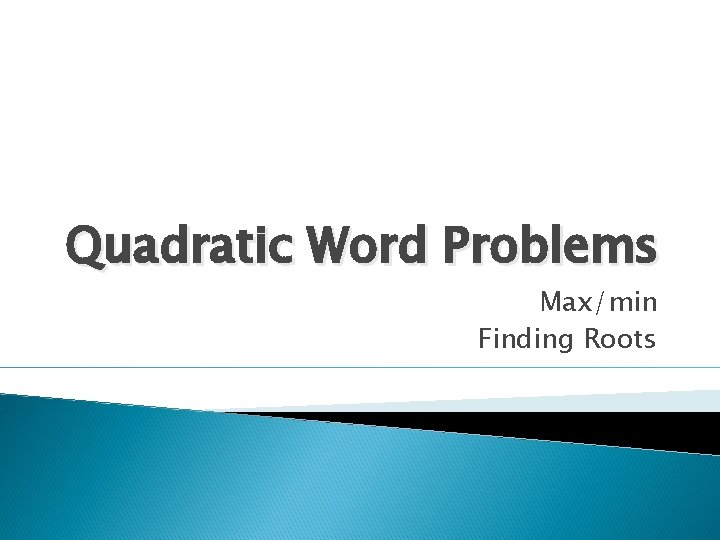Quadratic Word Problems Max/min Finding Roots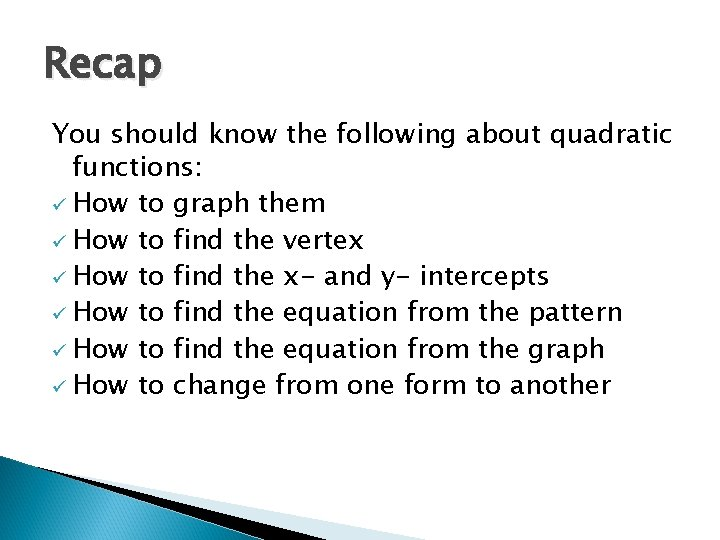Recap You should know the following about quadratic functions: ü How to graph them ü How to find the vertex ü How to find the x- and y- intercepts ü How to find the equation from the pattern ü How to find the equation from the graph ü How to change from one form to anotherQuadratic Word Problems There are basically two types of quadratic word problems: Those that ask you to find the vertex Those that ask you to find the roots By giving you the equationQuadratic Word Problems There are basically two types of quadratic word problems: Those that ask you to find the vertex Those that ask you to find the roots By giving you the information to find the equation These are the harder ones!!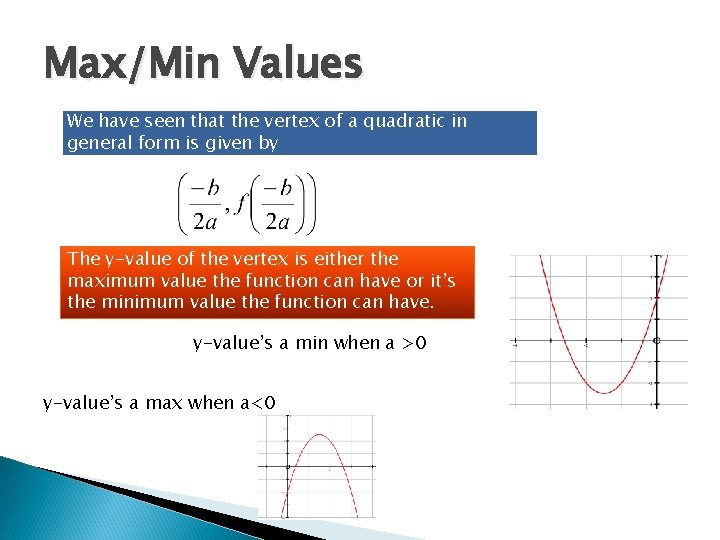Max/Min Values We have seen that the vertex of a quadratic in general form is given by The y-value of the vertex is either the maximum value the function can have or it’s the minimum value the function can have. y-value’s a min when a >0 y-value’s a max when a<0Max/Min Word Problems Example 1 A small business’ profits over the last year have been related to the price of the only product. The relationship is R(p) = -0. 4 p 2 +64 p-2400, where R is the revenue measured in thousands of dollars and p is the price of the product measured in dollars. a. What price would maximize the revenue? The word maximize screams “FIND THE VERTEX!!” Thus, a price of \$80 would maximize the revenue.Max/Min Word Problems Example 1 A small business’ profits over the last year have been related to the price of the only product. The relationship is R(p) = -0. 4 p 2 +64 p-2400, where R is the revenue measured in thousands of dollars and p is the price of the product measured in dollars. b. What is the maximum revenue possible? The answer to this question is the y-value of the vertex. The maximum revenue is \$160 000.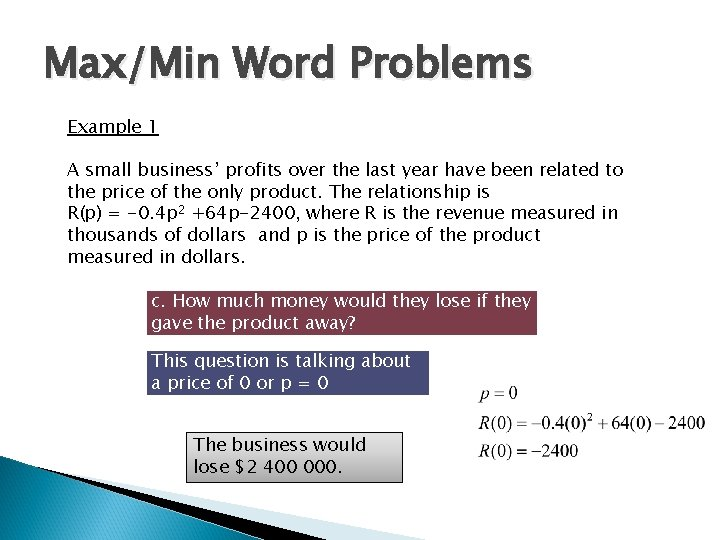Max/Min Word Problems Example 1 A small business’ profits over the last year have been related to the price of the only product. The relationship is R(p) = -0. 4 p 2 +64 p-2400, where R is the revenue measured in thousands of dollars and p is the price of the product measured in dollars. c. How much money would they lose if they gave the product away? This question is talking about a price of 0 or p = 0 The business would lose \$2 400 000.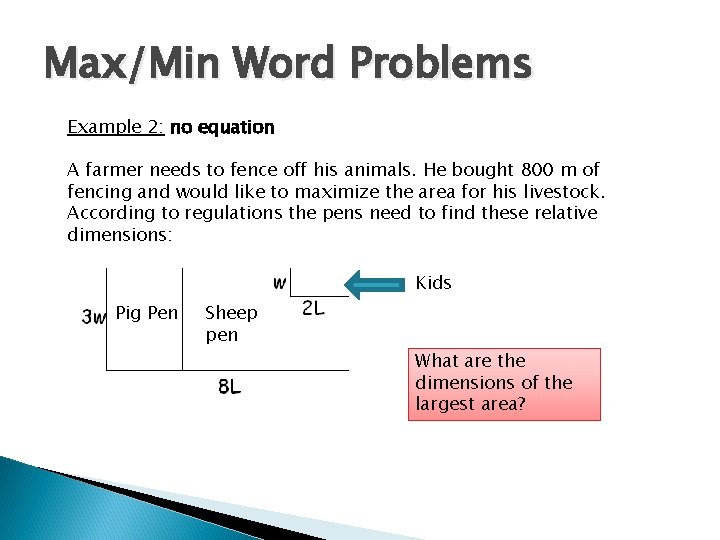Max/Min Word Problems Example 2: no equation A farmer needs to fence off his animals. He bought 800 m of fencing and would like to maximize the area for his livestock. According to regulations the pens need to find these relative dimensions: Kids Pig Pen Sheep pen What are the dimensions of the largest area?Max/Min Word Problems Example 2: no equation A farmer needs to fence off his animals. He bought 800 m of fencing and would like to maximize the area for his livestock. According to regulations the pens need to find these relative dimensions: Again the word Maximum means VERTEX. BUT… We need the equation!!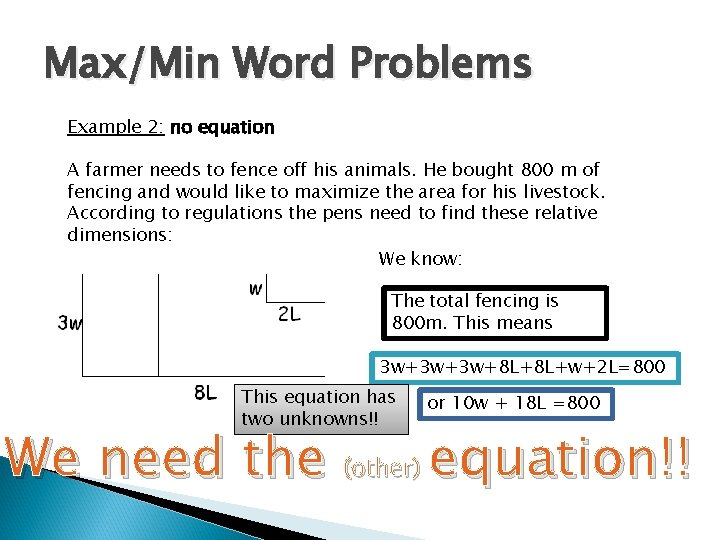Max/Min Word Problems Example 2: no equation A farmer needs to fence off his animals. He bought 800 m of fencing and would like to maximize the area for his livestock. According to regulations the pens need to find these relative dimensions: We know: The total fencing is 800 m. This means 3 w+3 w+3 w+8 L+8 L+w+2 L=800 This equation has two unknowns!! or 10 w + 18 L =800 We need the (other) equation!!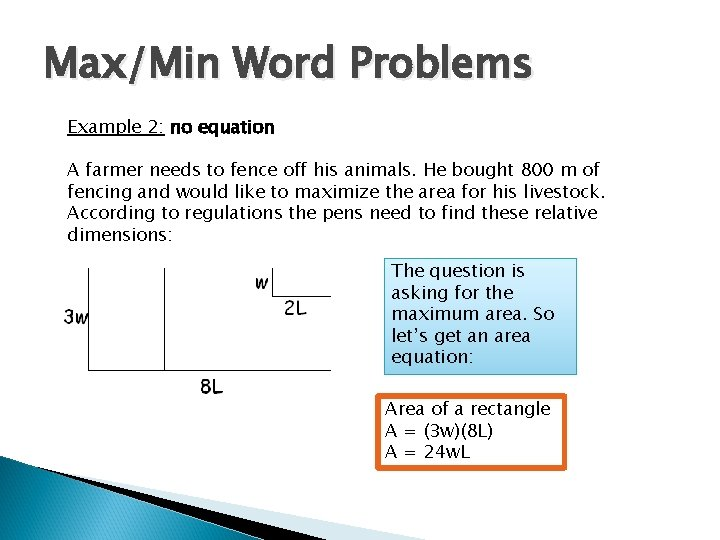Max/Min Word Problems Example 2: no equation A farmer needs to fence off his animals. He bought 800 m of fencing and would like to maximize the area for his livestock. According to regulations the pens need to find these relative dimensions: The question is asking for the maximum area. So let’s get an area equation: Area of a rectangle A = (3 w)(8 L) A = 24 w. L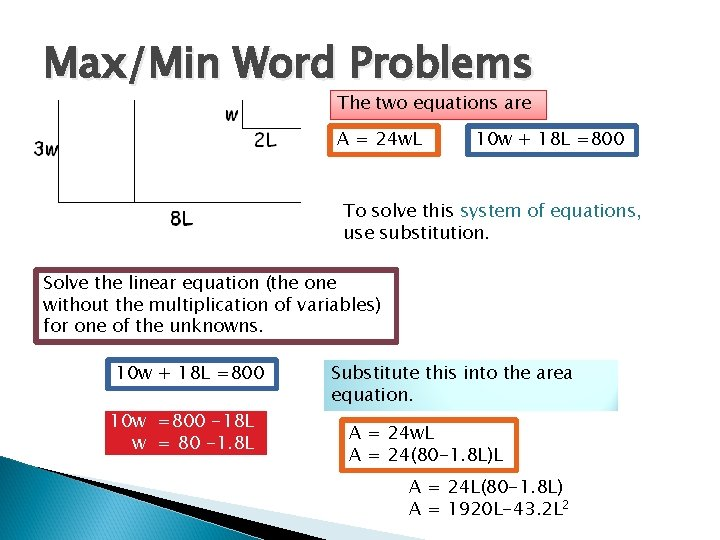Max/Min Word Problems The two equations are A = 24 w. L 10 w + 18 L =800 To solve this system of equations, use substitution. Solve the linear equation (the one without the multiplication of variables) for one of the unknowns. 10 w + 18 L =800 10 w =800 -18 L w = 80 -1. 8 L Substitute this into the area equation. A = 24 w. L A = 24(80 -1. 8 L)L A = 24 L(80 -1. 8 L) A = 1920 L-43. 2 L 2Max/Min Word Problems A = 1920 L-43. 2 L 2 Remember, we’re looking for the MAX AREA. We’ll find that with the vertex. The BEST L value to use is 22. 22 m This leads to a w value of w = 80 -1. 8 L w = 80 -1. 8(22. 22) w= 40. 00 mMax/Min Word Problems Example 2: no equation A farmer needs to fence off his animals. He bought 800 m of fencing and would like to maximize the area for his livestock. According to regulations the pens need to find these relative dimensions: The dimensions that would be the best are: w = 40. 00 m and L = 22. 22 m The largest area would be: A = 24 w. L A = 24(40. 00)(22. 22) A = 21331. 2 m 2Your Turn A lifeguard has 75 m of rope to section off the supervised area of the beach. What is the largest rectangular swimming area possible? 2 w + L = 75 A = w. L L= 75 -2 w A=w(75 -2 w) A = 75 w-2 w 2 A = 75(18. 75)-2(18. 75)2 A =1406. 25 -703. 125 A= 703. 125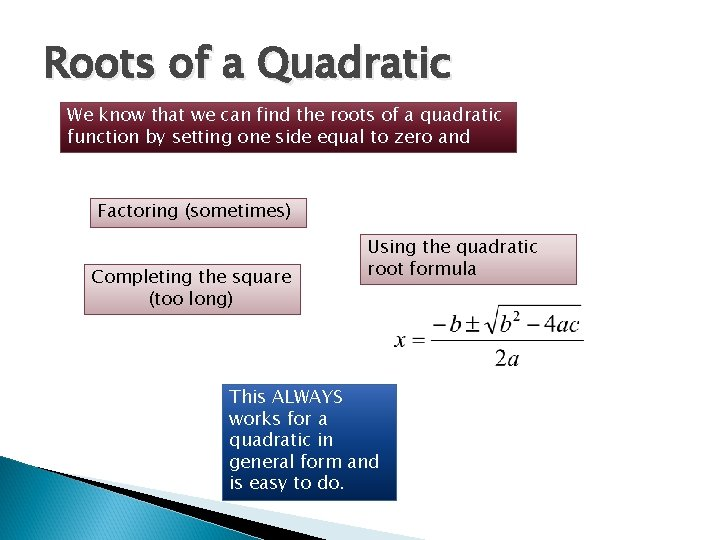Roots of a Quadratic We know that we can find the roots of a quadratic function by setting one side equal to zero and Factoring (sometimes) Completing the square (too long) Using the quadratic root formula This ALWAYS works for a quadratic in general form and is easy to do.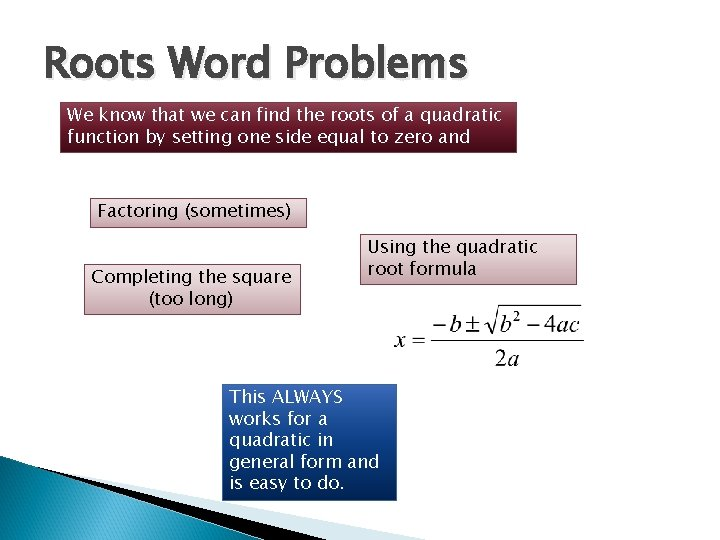Roots Word Problems We know that we can find the roots of a quadratic function by setting one side equal to zero and Factoring (sometimes) Completing the square (too long) Using the quadratic root formula This ALWAYS works for a quadratic in general form and is easy to do.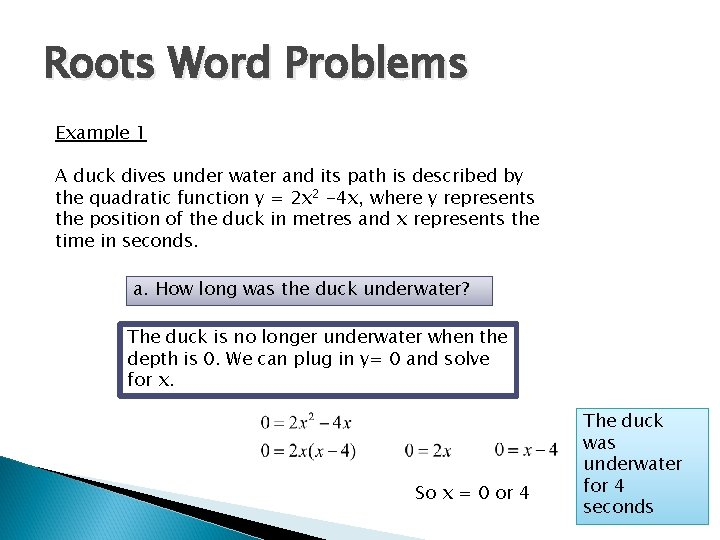Roots Word Problems Example 1 A duck dives under water and its path is described by the quadratic function y = 2 x 2 -4 x, where y represents the position of the duck in metres and x represents the time in seconds. a. How long was the duck underwater? The duck is no longer underwater when the depth is 0. We can plug in y= 0 and solve for x. So x = 0 or 4 The duck was underwater for 4 seconds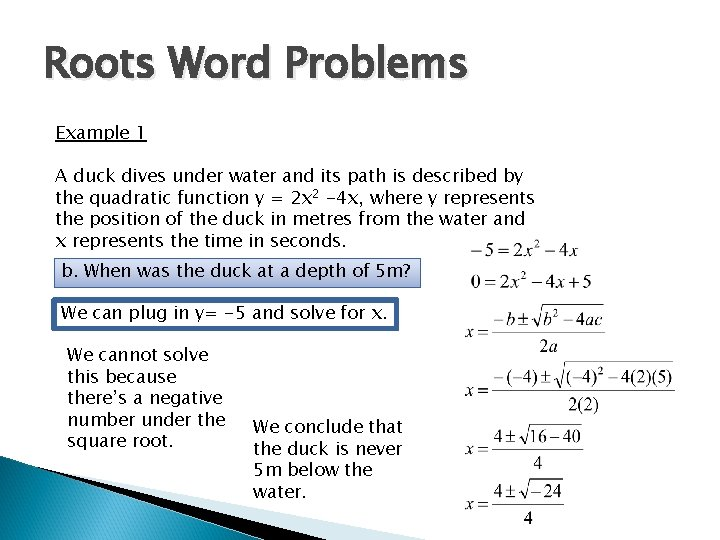Roots Word Problems Example 1 A duck dives under water and its path is described by the quadratic function y = 2 x 2 -4 x, where y represents the position of the duck in metres from the water and x represents the time in seconds. b. When was the duck at a depth of 5 m? We can plug in y= -5 and solve for x. We cannot solve this because there’s a negative number under the square root. We conclude that the duck is never 5 m below the water.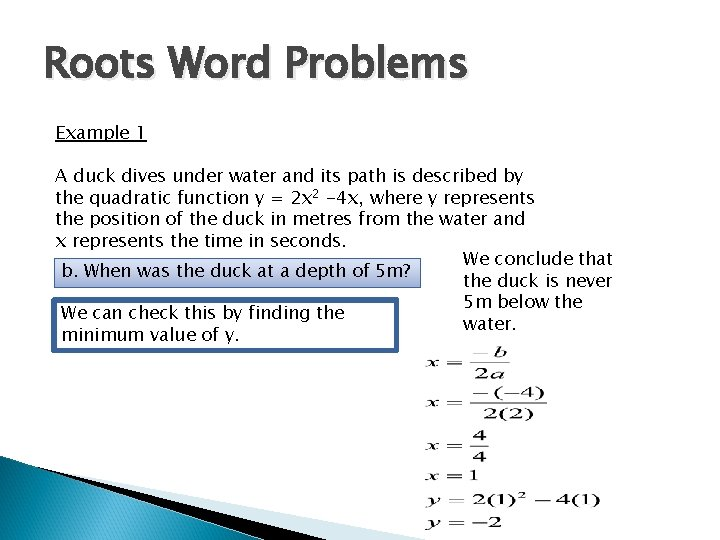Roots Word Problems Example 1 A duck dives under water and its path is described by the quadratic function y = 2 x 2 -4 x, where y represents the position of the duck in metres from the water and x represents the time in seconds. We conclude that b. When was the duck at a depth of 5 m? the duck is never 5 m below the We can check this by finding the water. minimum value of y.Roots Word Problems Example 1 A duck dives under water and its path is described by the quadratic function y = 2 x 2 -4 x, where y represents the position of the duck in metres and x represents the time in seconds. c. How long was the duck at least 0. 5 m below the water’s surface? We can plug in y= -0. 5 and solve for x. This will give us the times when the duck is at 0. 5 m below. The duck was 0. 5 m below at t = 0. 14 s and at t = 1. 87 s Therefore it was below 0. 5 m for 1. 73 s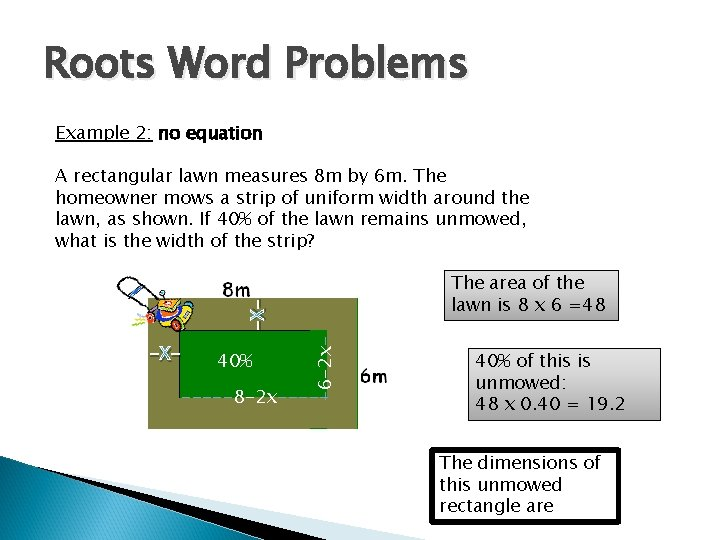Roots Word Problems Example 2: no equation A rectangular lawn measures 8 m by 6 m. The homeowner mows a strip of uniform width around the lawn, as shown. If 40% of the lawn remains unmowed, what is the width of the strip? -X- 40% -6 -2 x- -X- The area of the lawn is 8 x 6 =48 -----8 -2 x----- 40% of this is unmowed: 48 x 0. 40 = 19. 2 The dimensions of this unmowed rectangle areRoots Word Problems Example 2: no equation A rectangular lawn measures 8 m by 6 m. The homeowner mows a strip of uniform width around the lawn, as shown. If 40% of the lawn remains unmowed, what is the width of the strip? -X- 40% -6 -2 x- -X- So 19. 2 = (8 -2 x)(6 -2 x) -----8 -2 x----- We need to solve for x Make one side equal to 0 and use the quadratic root formula But first we FOIL it out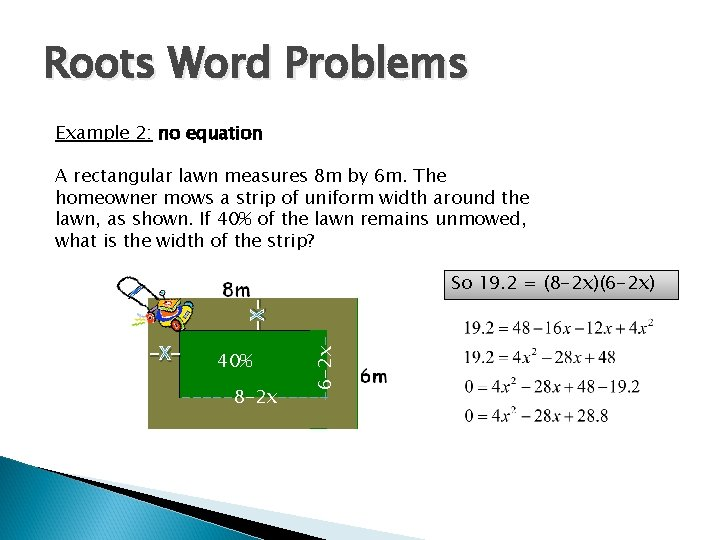Roots Word Problems Example 2: no equation A rectangular lawn measures 8 m by 6 m. The homeowner mows a strip of uniform width around the lawn, as shown. If 40% of the lawn remains unmowed, what is the width of the strip? -X- 40% -6 -2 x- -X- So 19. 2 = (8 -2 x)(6 -2 x) -----8 -2 x-----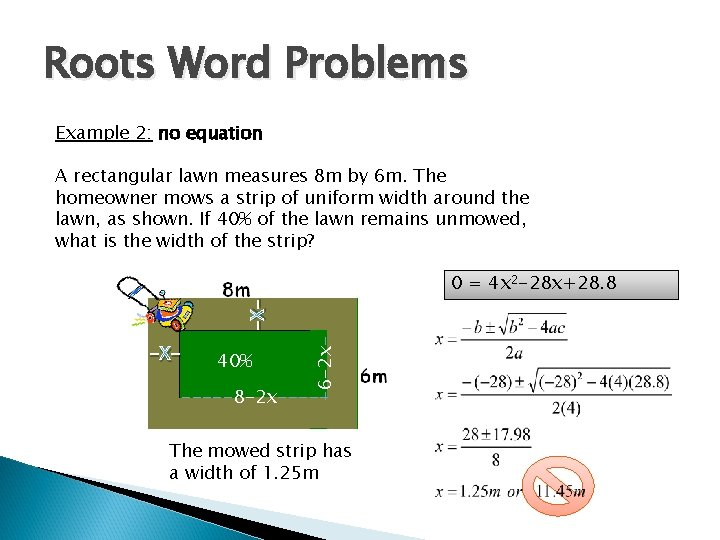Roots Word Problems Example 2: no equation A rectangular lawn measures 8 m by 6 m. The homeowner mows a strip of uniform width around the lawn, as shown. If 40% of the lawn remains unmowed, what is the width of the strip? -X- 40% -6 -2 x- -X- 0 = 4 x 2 -28 x+28. 8 -----8 -2 x----- The mowed strip has a width of 1. 25 m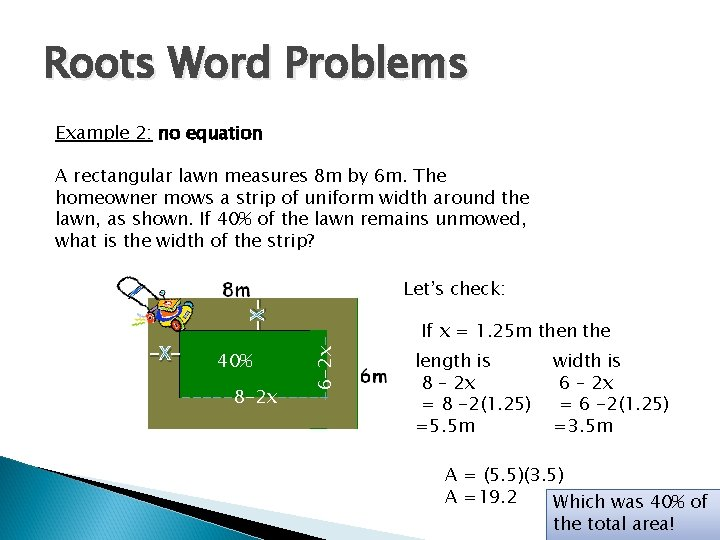Roots Word Problems Example 2: no equation A rectangular lawn measures 8 m by 6 m. The homeowner mows a strip of uniform width around the lawn, as shown. If 40% of the lawn remains unmowed, what is the width of the strip? -X- 40% -6 -2 x- -X- Let’s check: -----8 -2 x----- If x = 1. 25 m then the length is 8 – 2 x = 8 -2(1. 25) =5. 5 m width is 6 – 2 x = 6 -2(1. 25) =3. 5 m A = (5. 5)(3. 5) A =19. 2 Which was 40% of the total area!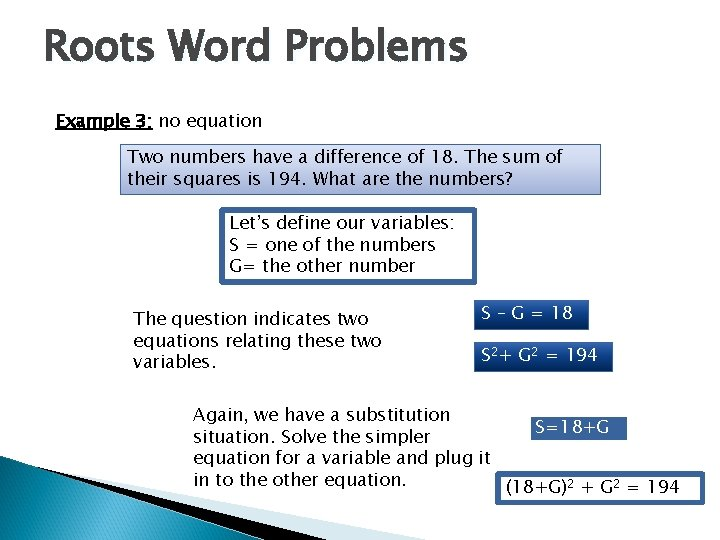Roots Word Problems Example 3: no equation Two numbers have a difference of 18. The sum of their squares is 194. What are the numbers? Let’s define our variables: S = one of the numbers G= the other number The question indicates two equations relating these two variables. S – G = 18 S 2+ G 2 = 194 Again, we have a substitution S=18+G situation. Solve the simpler equation for a variable and plug it in to the other equation. (18+G)2 + G 2 = 194Roots Word Problems Example 3: no equation Two numbers have a difference of 18. The sum of their squares is 194. What are the numbers? (18+G)2 + G 2 = 194 We need to solve this equation for G. Use the quadratic root formula Let’s FOIL and make one side equal to 0. 324+36 G+ G 2 = 194 2 G 2 +36 G+324 -194 =0 2 G 2 +36 G+130=0 S = 18 + (-5) = 13 or S = 18+(-13)=5Roots Word Problems Example 3: no equation Two numbers have a difference of 18. The sum of their squares is 194. What are the numbers? (18+G)2 + G 2 = 194 The numbers are either -5 and 13 or -13 and 5Roots Word Problems: Try one A rectangle is 8 feet long and 6 feet wide. If the same number of feet increases each dimension, the area of the new rectangle formed is 32 square feet more than the area of the original rectangle. How many feet increased each dimension? So 80 = (6+x)(8+x) 80=48+14 x+x 2 0=x 2 +14 x-32 x =2 or x = -16 The new are is 32+6 x 8 =32+48 =80 The dimensions of the new rectangle are 6+x and 8+xMay I have a Word (Problem) A ball is thrown and follows the path described by the function h(t) = -5 t 2 +20 t +1, where h is the height of the ball and t is the time since the ball was released. a) When was the ball at a height of 3. 5 m? This question is looking for ‘t’ so it gives a specific h. In this case h = 3. 5 = -5 t 2 +20 t +1 We will solve this by setting one side equal to zero and using the quadratic root formula One time is on the way up and the other is on the way down. 0 = -5 t 2 +20 t -2. 5May I have a Word (Problem) A ball is thrown and follows the path described by the function h(t) = -5 t 2 +20 t +1, where h is the height of the ball and t is the time since the ball was released. a) When was the ball at a height of 3. 5 m? The ball is at a height of 3. 5 m at two times: at t= 0. 129 s and at t = 3. 871 s 0 = -5 t 2 +20 t -2. 5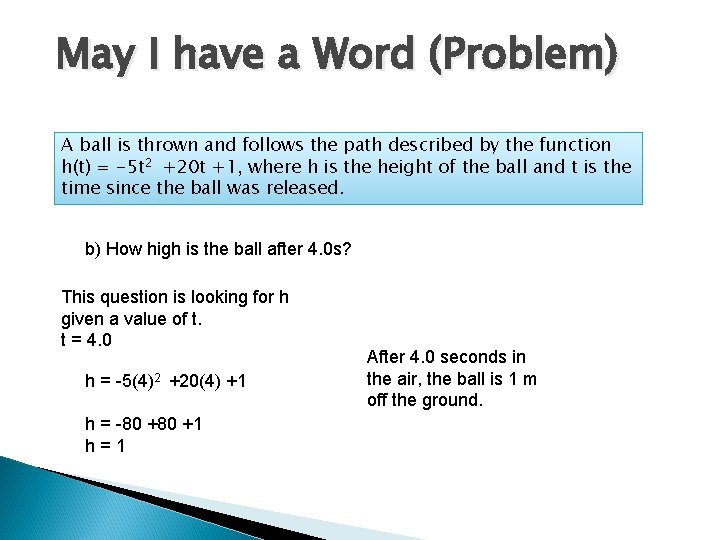May I have a Word (Problem) A ball is thrown and follows the path described by the function h(t) = -5 t 2 +20 t +1, where h is the height of the ball and t is the time since the ball was released. b) How high is the ball after 4. 0 s? This question is looking for h given a value of t. t = 4. 0 h = -5(4)2 +20(4) +1 h = -80 +1 h=1 After 4. 0 seconds in the air, the ball is 1 m off the ground.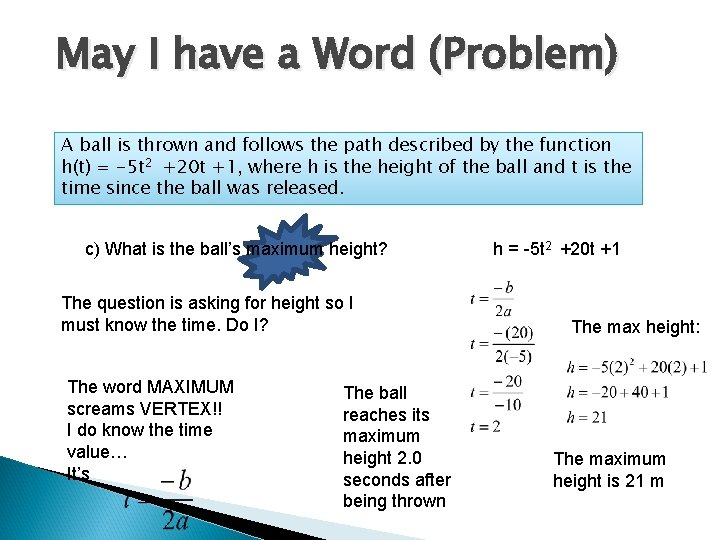May I have a Word (Problem) A ball is thrown and follows the path described by the function h(t) = -5 t 2 +20 t +1, where h is the height of the ball and t is the time since the ball was released. c) What is the ball’s maximum height? The question is asking for height so I must know the time. Do I? The word MAXIMUM screams VERTEX!! I do know the time value… It’s The ball reaches its maximum height 2. 0 seconds after being thrown h = -5 t 2 +20 t +1 The max height: The maximum height is 21 mMay I have a Word (Problem) A ball is thrown and follows the path described by the function h(t) = -5 t 2 +20 t +1, where h is the height of the ball and t is the time since the ball was released. d) When does the ball hit the ground? This question is asking for the time so I must know the height. The height is 0 – hitting the ground! Time can’t be negative so this cannot be an answer. 0 = -5 t 2 +20 t +1May I have a Word (Problem) A ball is thrown and follows the path described by the function h(t) = -5 t 2 +20 t +1, where h is the height of the ball and t is the time since the ball was released. e) From what height was the ball thrown? This question is asking for the height so I must know the time. The time is 0 – just before it is thrown! h = -5(0)2 +20(0)+1 h =1